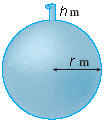# Work: Spherical Problem

RedBarchetta
[SOLVED] Work: Spherical Problem

## Homework Statement

A tank is full of water. Find the work W required to pump the water out of the spout. (Use 9.8 for g and 3.14 for [PLAIN]http://www.webassign.net/images/pi.gif.[/URL] [Broken] Round your answer to three significant digits.) W=_________

r=3
h=1## The Attempt at a Solution

To set up this problem, I started by taking the area of one slice of water to be pi r$$^{2}$$ multiplied by an infitesimally small height $$\Delta y$$ to get volume. Then multiply this by the density of water; 1000 kg/m$$^{3}$$ to get the volume of one slice.

Now I want R as a function of, lets say y. We know the area of a circle is r$$^{2}$$2+y$$^{2}$$=3$$^{2}$$. So r=sqrt(9-y$$^{2}$$). Also, the distance for any slice from the top is 7-y.

After this I tried integrating from zero to six of the function 1000*pi*(9-y$$^{2}$$)*(7-y)*y. This came out to be negative....in fact -36000*pi..

Apparently, that is wrong. Where did I go wrong?

Thanks.

Last edited by a moderator:

Homework Helper
Gold Member
Your expression for R as a function of y is wrong.

RedBarchetta
The above equation was really meant to be r$$^{2}$$+y$$^{2}$$=3$$^{2}$$. I just noticed the typo. Or, are you saying that's wrong?

Homework Helper
Be careful about what y represents. $r= \sqrt{9- y^2}$ is correct if is the height above the center of the sphere. But in that case, integrating from 0 to 6 is wrong. Integrating from the bottom of the tank to the top takes y from -3 to 3.

Last edited by a moderator:
RedBarchetta
I integrated and got 2469600*pi as my answer. My homework checker told me I was wrong. I even rounded to the proper amount of sig figs(3).

Heres what I have:
dA=(9-y$$^{2}$$)pi
(Multiplied by infitesimally thickness dy)
dV=(9-y$$^{2}$$)pi dy
(Since d=mv, I multiplied density of water (1000kg/m$$^{3}$$)
dM=1000(9-y$$^{2}$$)pi dy
(multiplied by gravity 9.8 m/s$$^{2}$$)
dF=9800(9-y$$^{2}$$)pi dy
(Now multiplied by distance (7-y))
dW=9800(9-y$$^{2}$$)(7-y) dy

Now I integrated dW with the limits from -3 to 3 and got the above result. I'm lost.Thanks for the help Ivy.

Homework Helper
Gold Member
The distance to the top would be 7 - y if you chose y from the bottom. It appears that you have chosen y as the distance from the center.

RedBarchetta
Great! 4-y worked.

I can finally put this problem to rest. Thanks for the help everyone!

Last edited: